# The cube root of 1331 times the cube root of 64 is

Welcome to Catalytic, a blog to discuss the discussion of subject matter from Higher Education, High School, Middle School and Elementary Schools. This time Katalistiwa will discuss a question that is often asked in school exams. The questions are: The cube root of 1331 times the cube root of 64 is

## The cube root of 1331 times the cube root of 64 is

Result of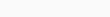is 44. The cube root of 1.331 is 11. The cube root of 64 is 4.

### Detailed discussion of Root 3

CUBE ROOT

Finding the cube root can be done by:

1. Create a factor tree from number the.
2. Write down the factorization of the number.
3. Divide the factorization power of a number by 3.

Example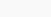1.728

/   \\

2    864

/   \\

2    432

/   \\

2    216

/   \\

2   108

/   \\

2    54

/   \\

2    27

/  \\

3    9

/  \\

3   3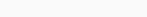=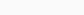=2² × 3¹

= 4×3

=12?

Explanation

Determine1.331

/  \\

11   121

/  \\

11   11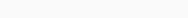= 11¹ = 11

Determine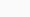64

/  \\

2   32

/   \\

2    16

/  \\

2    8

/  \\

2    4

/  \\

2   2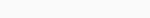= 2² = 4=11 × 4

=44

Question Details

Class : VI

Course : Mathematics

Chapter : Cubic and Cube Roots

This is the discussion that we have summarized by the Katalistiwa.id Team from various learning sources. Hopefully this discussion is useful, don't forget if you have other answers, you can contact the admin. Thank you